Filter By

## All Questions

#### A point particle of mass m, moves along the uniformly rough track PQR as shown in the figure.  The coefficient of friction, between the particle and the rough track equals µ.  The particle is released, from rest, from the point P and it comes to rest at a point R.  The energies, lost by the ball, over the parts, PQ and QR, of the track, are equal to each other, and no energy is lost when particle changes direction from PQ to QR. The values of the coefficient of friction µ and the distance x(=QR), are, respectively close to :Option: 1  0.2 and 6.5 m   Option: 3  0.2 and 3.5 m   Option: 4   0.29 and 6.5 m

Work done by friction at QR = μmgx

In triangle, sin 30° = 1/2 = 2/PQ

PQ = 4 m

Work done by friction at PQ = μmg × cos 30° × 4 = μmg × √3/2 × 4 = 2√3μmg

Since work done by friction on parts PQ and QR are equal,

μmgx = 2√3μmg

x = 2√3 ≅ 3.5 m

Applying work energy theorem from P to R

decrease in P.E.=P.E.= loss of energy due to friction in PQPQ and QR

where h=2(given)

#### A particle of mass m is moving along the side of a square of side ‘a’, with a uniform speedin the x-y plane as shown in the figure :Which of the following statements is false for the angular momentumabout the origin? Option: 1 when the particle is moving from A to B. Option: 2 when the particle is moving from C to D. Option: 3 when the particle is moving from B to C. Option: 4when the particle is moving from D to A.

so option b is correct option

## Crack CUET with india's "Best Teachers"

• HD Video Lectures
• Unlimited Mock Tests
• Faculty Support#### A body of mass m=10−2 kg is moving in a medium and experiences a frictional forceIts initial speed is.  If, after 10 s, its energy isthe value of k will be :   Option: 1 10−3 kg m−1   Option: 2  10−3 kg s−1   Option: 3  10−4 kg m−1   Option: 4 10−1 kg m−1 s−1

As we learnt in#### Consider a uniform rod of mass and length l pivoted about its centre. A mass m moving with velocity  making angle  to the rod's long axis collides with one end of the rod and sticks to it. The angular speed of the rod - mass system just after the collision is :    Option: 1Option: 2Option: 3Option: 4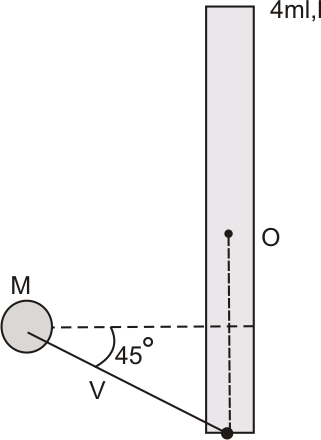Let us conserve angular momentum about O:-

So, , where  is linear momentum and  is the distance from centre O.

Now,

Here,

So,

So the correct graph is given in option 2.

## Crack NEET with "AI Coach"

• HD Video Lectures
• Unlimited Mock Tests
• Faculty Support#### A particle of mass m is fixed to one end of a light spring having force constant k and unstretched length l. The other end is fixed. The system is given an angular speedabout the fixed end of the spring such that it rotates in a circle in gravity-free space. Then the stretch in the spring is :      Option: 1Option: 2Option: 3Option: 4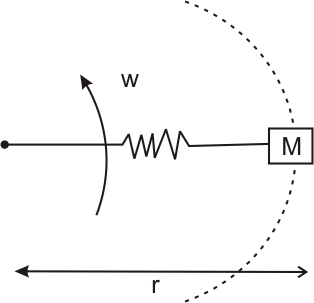As natural lentgh=l

Let elongation=x

Mass m is moving with angular velocityin a radius r

whereDue to elongation x spring force is given byAndasSoSo the correct option is 2.

####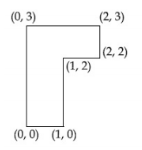The coordinates of centre of mass of a uniform flag shaped lamina (thin flat plate) of mass. (The coordinates of the same are shown in the figure) are : Option: 1Option: 2Option: 3Option: 4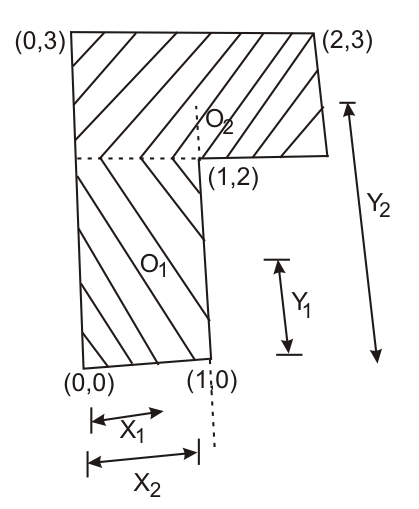The co-ordinate of O1 is (0.5,1),  O2 is (1, 2.5)So the correct option is 3.

## Crack JEE Main with "AI Coach"

• HD Video Lectures
• Unlimited Mock Tests
• Faculty Support#### A spring mass system (mass m, spring constant k and natural length l ) rests in equilibrium on a horizontal disc.The free end of the spring is fixed at the centre of the disc. If the disc together with spring mass system, rotates about its axis with an angular velocity ,  the relative change in the length of the spring is best given by the option : Option: 1Option: 2Option: 3Option: 4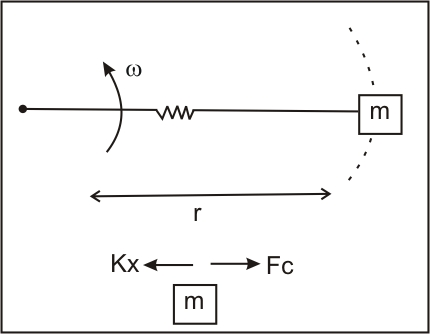As natural lentgh=l0

Let elongation=x

Mass m is moving with angular velocity in a radius r

where

Due to elongation x spring force is given by

And

as

Using

So,  is equal to

Hence the correct option is (2).

#### Three point particles of masses 1.0 kg, 1.5 kg and 2.5 kg are placed at three corners of a right triangle of sides 4.0 cm, 3.0 cm, and 5.0 cm as shown in the figure. The centre of mass of the system is at a point: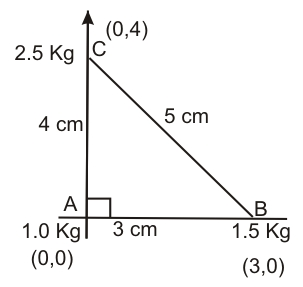Option: 1right andabovemass     Option: 2right andabovemass Option: 3right andabovemass   Option: 4right andabovemasslet m1=1 kg, m2=1.5 kg and m3=2.5 kg

x1=0, x2=3, x3=0 and y1=0, y2=0, y3=4andLet point A be origin and mass m1=1.0 kg be at origin.

So,andso centre of mass of the system is at (0.9,2).

So from the figure we can say that the 0.9 cm right and 2 cm above the 1 kg mass.

So option (2) is correct.

## Crack CUET with india's "Best Teachers"

• HD Video Lectures
• Unlimited Mock Tests
• Faculty Support#### As shown in the figure, a bob of mass m is tied by a massless string whose other end portion is wound on a flywheel (disc) of radius r and mass m. When released from rest the bob starts falling vertically. When it has covered a distance h, the angular speed of the wheel will be:-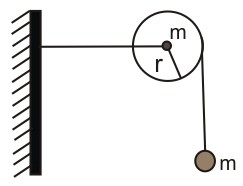Option: 1Option: 2Option: 3Option: 4Conservation Of angular momentum -Hence option (3) is correct.

#### The radius of gyration of a uniform rod of length l, about an axis passing through a pointaway from the centre of the rod and perpendicular to it is:-   Option: 1Option: 2Option: 3Option: 4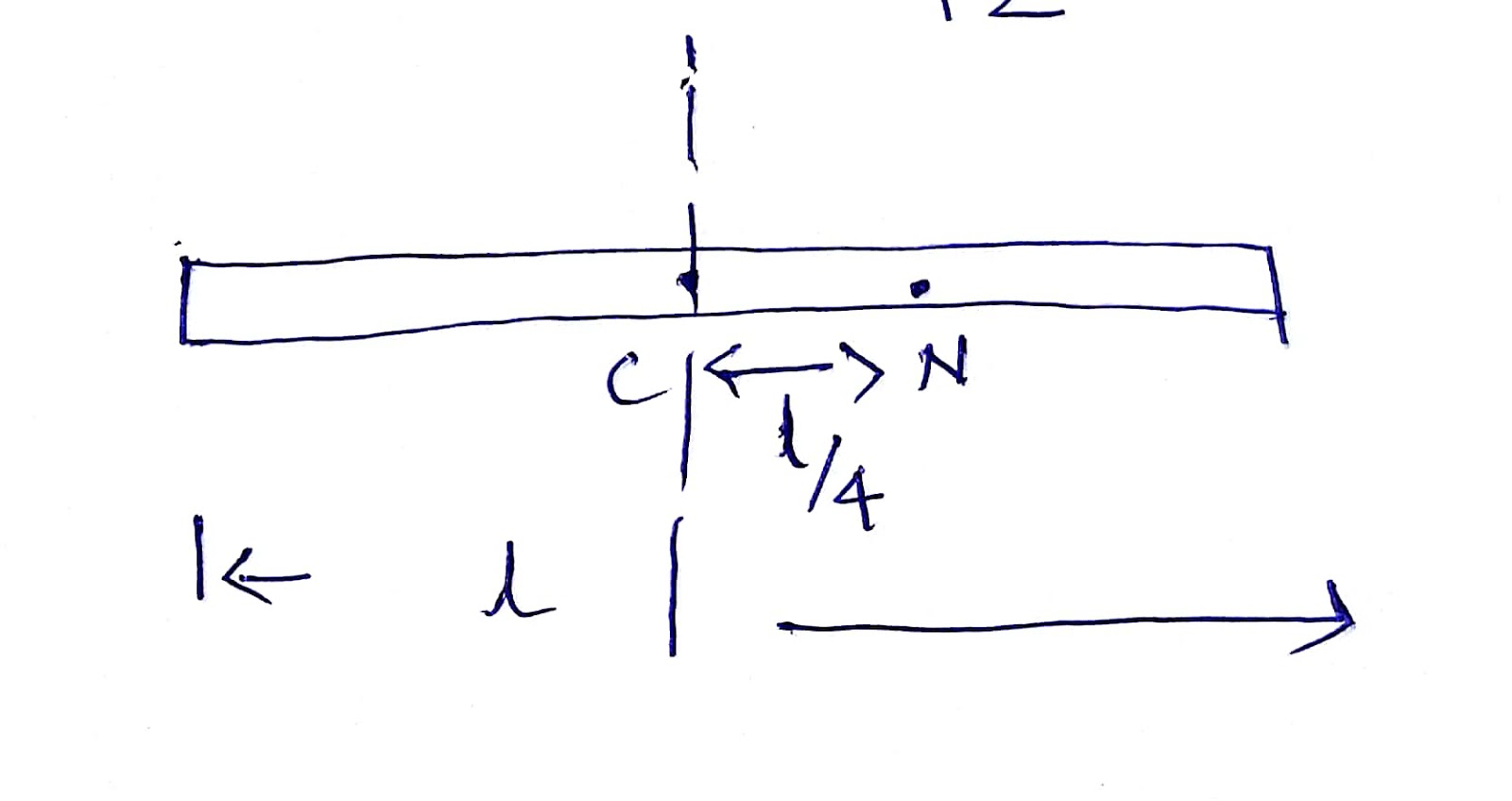Moment of inertia of rod about an axis perpendicular through COMAndSo the option (2) is correct.